# lecture 1 Slides.pptx

May. 28, 2023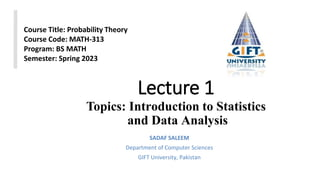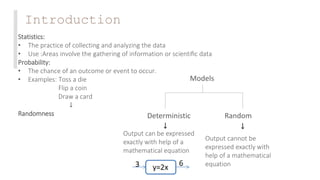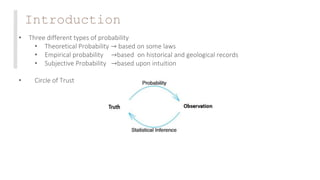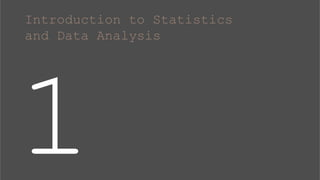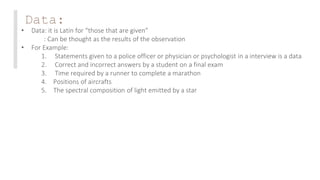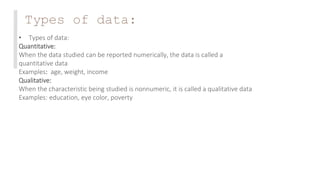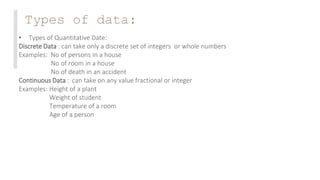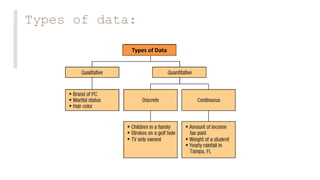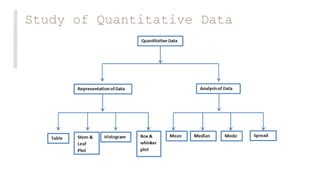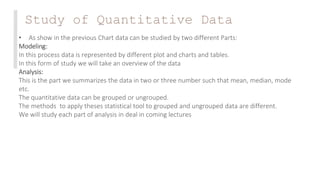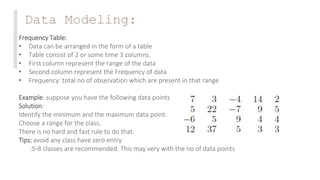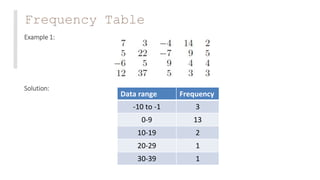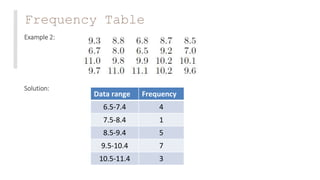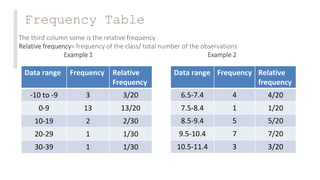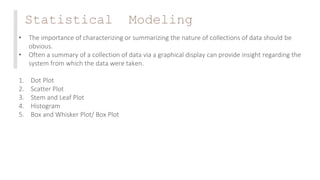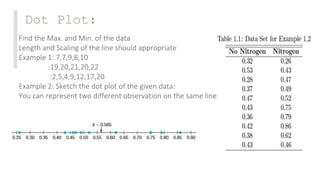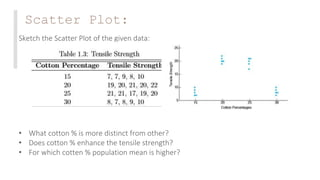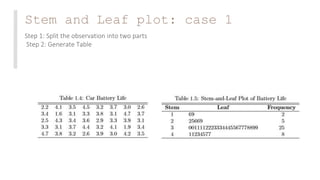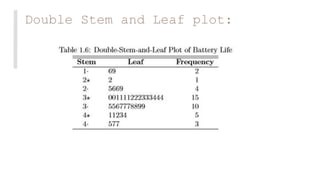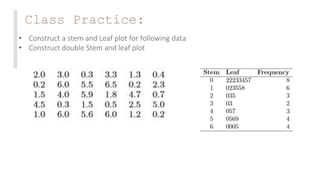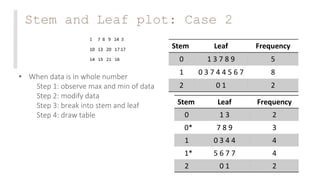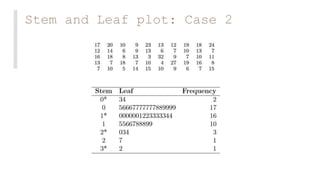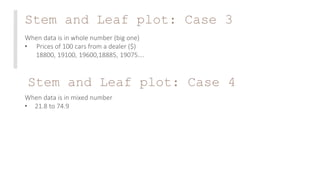1 of 24

### lecture 1 Slides.pptx

• 1. Lecture 1 Topics: Introduction to Statistics and Data Analysis SADAF SALEEM Department of Computer Sciences GIFT University, Pakistan Course Title: Probability Theory Course Code: MATH-313 Program: BS MATH Semester: Spring 2023
• 2. Introduction Statistics: • The practice of collecting and analyzing the data • Use :Areas involve the gathering of information or scientiﬁc data Probability: • The chance of an outcome or event to occur. • Examples: Toss a die Flip a coin Draw a card ↓ Randomness Deterministic Random Models ↓ ↓ Output can be expressed exactly with help of a mathematical equation Output cannot be expressed exactly with help of a mathematical equation
• 3. Introduction • Three different types of probability • Theoretical Probability → based on some laws • Empirical probability →based on historical and geological records • Subjective Probability →based upon intuition • Circle of Trust
• 5. Data: • Data: it is Latin for “those that are given” : Can be thought as the results of the observation • For Example: 1. Statements given to a police officer or physician or psychologist in a interview is a data 2. Correct and incorrect answers by a student on a final exam 3. Time required by a runner to complete a marathon 4. Positions of aircrafts 5. The spectral composition of light emitted by a star
• 6. Types of data: • Types of data: Quantitative: When the data studied can be reported numerically, the data is called a quantitative data Examples: age, weight, income Qualitative: When the characteristic being studied is nonnumeric, it is called a qualitative data Examples: education, eye color, poverty
• 7. Types of data: • Types of Quantitative Date: Discrete Data : can take only a discrete set of integers or whole numbers Examples: No of persons in a house No of room in a house No of death in an accident Continuous Data : can take on any value fractional or integer Examples: Height of a plant Weight of student Temperature of a room Age of a person
• 10. Study of Quantitative Data • As show in the previous Chart data can be studied by two different Parts: Modeling: In this process data is represented by different plot and charts and tables. In this form of study we will take an overview of the data Analysis: This is the part we summarizes the data in two or three number such that mean, median, mode etc. The quantitative data can be grouped or ungrouped. The methods to apply theses statistical tool to grouped and ungrouped data are different. We will study each part of analysis in deal in coming lectures
• 11. Data Modeling: Frequency Table: • Data can be arranged in the form of a table • Table consist of 2 or some time 3 columns. • First column represent the range of the data • Second column represent the Frequency of data • Frequency: total no of observation which are present in that range Example: suppose you have the following data points Solution: Identify the minimum and the maximum data point. Choose a range for the class, There is no hard and fast rule to do that. Tips: avoid any class have zero entry :5-8 classes are recommended. This may very with the no of data points
• 12. Frequency Table Example 1: Solution: Data range Frequency -10 to -1 3 0-9 13 10-19 2 20-29 1 30-39 1
• 13. Frequency Table Example 2: Solution: Data range Frequency 6.5-7.4 4 7.5-8.4 1 8.5-9.4 5 9.5-10.4 7 10.5-11.4 3
• 14. Frequency Table The third column some is the relative frequency Relative frequency= frequency of the class/ total number of the observations Example 1 Example 2 Data range Frequency Relative Frequency -10 to -9 3 3/20 0-9 13 13/20 10-19 2 2/30 20-29 1 1/30 30-39 1 1/30 Data range Frequency Relative frequency 6.5-7.4 4 4/20 7.5-8.4 1 1/20 8.5-9.4 5 5/20 9.5-10.4 7 7/20 10.5-11.4 3 3/20
• 15. Statistical Modeling • The importance of characterizing or summarizing the nature of collections of data should be obvious. • Often a summary of a collection of data via a graphical display can provide insight regarding the system from which the data were taken. 1. Dot Plot 2. Scatter Plot 3. Stem and Leaf Plot 4. Histogram 5. Box and Whisker Plot/ Box Plot
• 16. Dot Plot: Find the Max. and Min. of the data Length and Scaling of the line should appropriate Example 1: 7,7,9,8,10 :19,20,21,20,22 :2,5,4,9,12,17,20 Example 2: Sketch the dot plot of the given data: You can represent two different observation on the same line
• 17. Scatter Plot: Sketch the Scatter Plot of the given data: • What cotton % is more distinct from other? • Does cotton % enhance the tensile strength? • For which cotten % population mean is higher?
• 18. Stem and Leaf plot: case 1 Step 1: Split the observation into two parts Step 2: Generate Table
• 19. Double Stem and Leaf plot:
• 20. Class Practice: • Construct a stem and Leaf plot for following data • Construct double Stem and leaf plot
• 21. Stem and Leaf plot: Case 2 • When data is in whole number Step 1: observe max and min of data Step 2: modify data Step 3: break into stem and leaf Step 4: draw table Stem Leaf Frequency 0 1 3 7 8 9 5 1 0 3 7 4 4 5 6 7 8 2 0 1 2 Stem Leaf Frequency 0 1 3 2 0* 7 8 9 3 1 0 3 4 4 4 1* 5 6 7 7 4 2 0 1 2
• 22. Stem and Leaf plot: Case 2
• 23. Stem and Leaf plot: Case 3 When data is in whole number (big one) • Prices of 100 cars from a dealer (\$) 18800, 19100, 19600,18885, 19075…. When data is in mixed number • 21.8 to 74.9 Stem and Leaf plot: Case 4